×# Maya材质贴图之四则运算节点知识介绍

Hello，大家好，今天给大家带来Maya材质贴图之四则运算节点知识介绍，我是几维。1、加法、减法、乘法和除法统称四则运算。

2、没有括号的算式，如果只有加、减法或者只有乘、除法，从左往右按顺序计算。

3、没有括号的算式，既有乘、除法又有加、减法的，要先算乘除法，再算加减法。

4、算式有括号，先算括号里面，再算括号外面。

5、0不能做除数。

6、一个数加上0还得原数。

7、一个数减去0还得原数。

8、一个数减去它本身，差是0。

9、一个数和0相乘，仍得0。

10、0除以任何非0的数，还得0。

Multiply

0=黑色，1=白色。所以通过这两个节点相乘得到的黑白图是这个结果。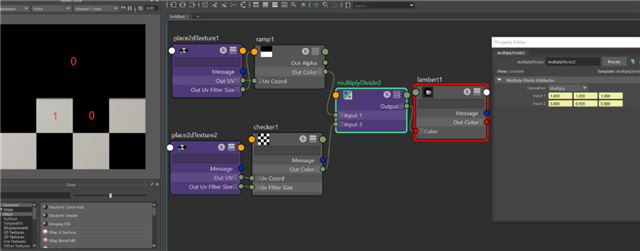Divide

0=黑色，1=白色。所以通过这两个节点相除得到的黑白图是这个结果。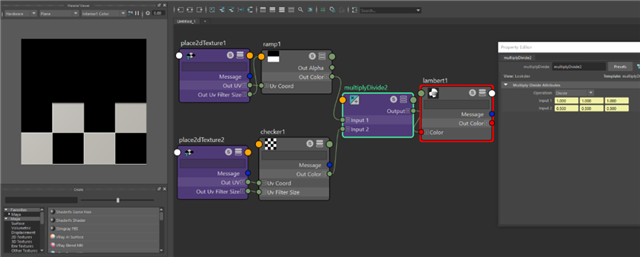0=黑色，1=白色，0.5=中间灰色。所以通过这两个节点相乘得到的黑白图是这个结果。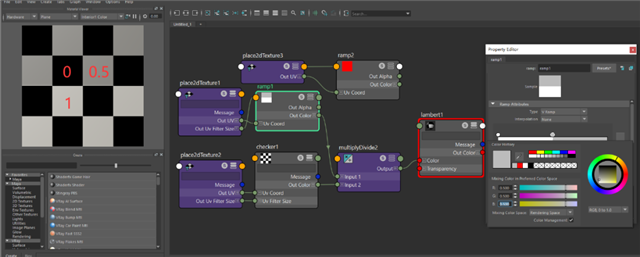Lambert材质球 Transparency参数黑色为不透明 白色为透明。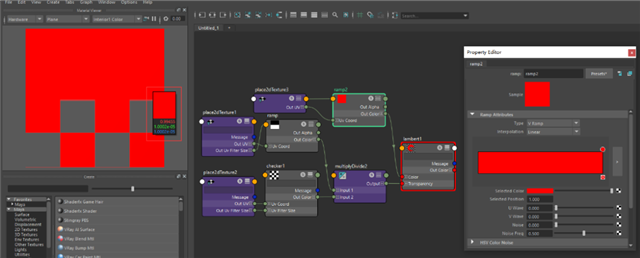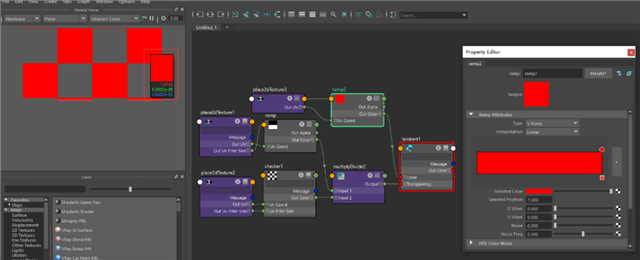Plus

(0,0,0)=RGB就代表着3维向量接连Input3D和output3D。

1+0=1, 0+1=1, 0+0=0。

(1,0,0)红+(0,1,0)绿=(1,1,0)黄。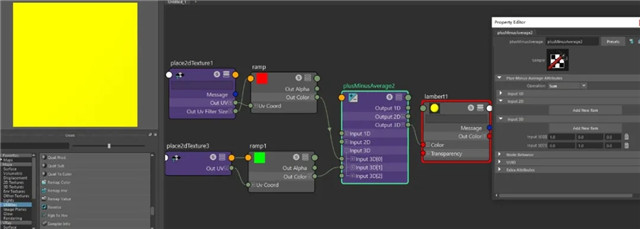1+0=1,0+0=0,0+1=1。

(1,0,0)红+(0,0,1)蓝=(1,0,1)紫。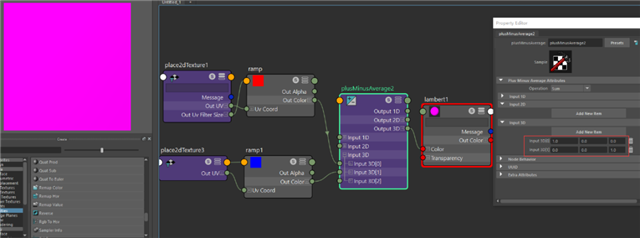Minus

(0,0,0)=RGB就代表着3维向量接连Input3D和output3D。

(1,0,0)=红色 (0,1,0)=绿色 (0,0,1)=蓝色。

1-0=1, 0-0=0, 0-1=-1

(在maya plusMinusAverage节点中不会计算出负数,默认都归为0)。

(1,0,0)红-(0,0,1)蓝=(1,0,0)红。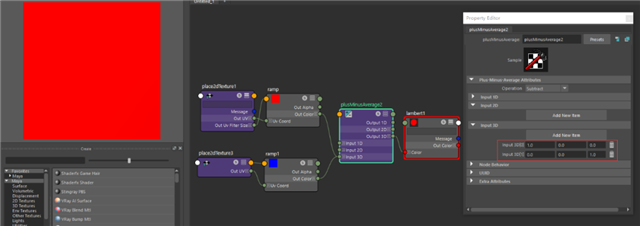1903学员阮捍、徐珂小组作品1903学员周德志小组作品1903学员周德志小组作品1903学员王悦、王思琪小组作品1903学员王悦、王思琪小组作品1903期学员阮捍、徐珂小组作品1903期学员阮捍、徐珂小组作品1903期学员阮捍、徐珂小组作品